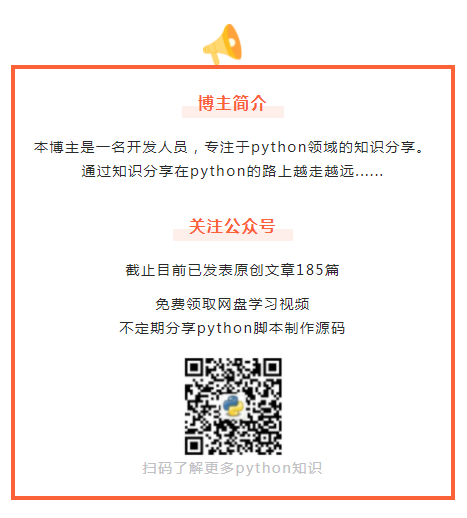出租广告位,需要合作请联系站长

+关注

## python几个常见的数据处理操作，一行代码就能完成！【阅读全文】

1、如何统一列表中的元素对象的类型？

``````list_1 = ['a', 1, 3.0, 2, '1']
``````

``````list_2 = list(map(str, list_1))

print(list_2)
``````

``````['a', '1', '3.0', '2', '1']
``````

2、如何将子列表的元素全部合并到父列表中？

``````list_3 = [['a', 'b'], ['c'], ['d', 'e', 'f'], ['g']]
``````

``````list_4 = ['a', 'b', 'c', 'd', 'e', 'f', 'g']
``````

``````list_5 = [item for list_ in list_3 for item in list_]

print(list_5)

# ['a', 'b', 'c', 'd', 'e', 'f', 'g']
``````

3、如何交换一个字典中的键值对？就是将键：值反过来。

``````dic_1 = {'name': 'Python 集中营', 'age': '1', 'sex': 'man'}

dic_2 = {a: b for b, a in dic_1.items()}  # 通过直接遍历出键:值，同时键:进行交换。

print(dic_2)
``````

``````{'Python 集中营': 'name', '1': 'age', 'man': 'sex'}
``````

4、如何使用map()函数计算所有整数位之和？

``````比如：123 = 1 + 2 + 3 = 6,511 = 5 + 1 + 1 = 7;
``````

``````print(sum([4, 5, 6]))
# 15
``````

``````list10 = list(map(int,str(456)))
print(list10)
# [4, 5, 6]
``````

``````sum(map(int,str(456)))
``````

``````func_sum = lambda a: sum(map(int,str(a)))

print(func_sum(7891))  # 调用该函数

# 得到结果 25
``````【往期精彩】

PyQt5 最小化到托盘，升级小闹钟...

PyQt5 小工具：Excel数据分组汇总器...

1 0

pdf(new) 更多>